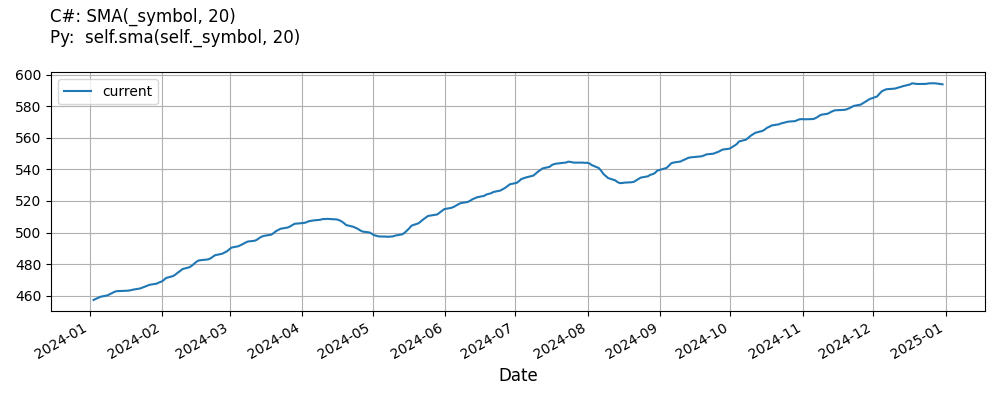# Supported Indicators

## Simple Moving Average

### Introduction

This indicator represents the traditional simple moving average indicator (SMA)

To view the implementation of this indicator, see the LEAN GitHub repository.

### Using SMA Indicator

To create an automatic indicators for SimpleMovingAverage, call the SMA helper method from the QCAlgorithm class. The SMA method creates a SimpleMovingAverage object, hooks it up for automatic updates, and returns it so you can used it in your algorithm. In most cases, you should call the helper method in the Initialize method.

public class SimpleMovingAverageAlgorithm : QCAlgorithm
{
private Symbol _symbol;
private SimpleMovingAverage _sma;

public override void Initialize()
{
_sma = SMA(_symbol, 20);
}

public override void OnData(Slice data)
{
{
// The current value of _sma is represented by itself (_sma)
// or _sma.Current.Value
Plot("SimpleMovingAverage", "sma", _sma);
// Plot all properties of sma
Plot("SimpleMovingAverage", "rollingsum", _sma.RollingSum);
}
}
}
class SimpleMovingAverageAlgorithm(QCAlgorithm):
def Initialize(self) -> None:
self.sma = self.SMA(self.symbol, 20)

def OnData(self, slice: Slice) -> None:
# The current value of self.sma is represented by self.sma.Current.Value
self.Plot("SimpleMovingAverage", "sma", self.sma.Current.Value)
# Plot all attributes of self.sma
self.Plot("SimpleMovingAverage", "rollingsum", self.sma.RollingSum.Current.Value)


The following reference table describes the SMA method:

### SMA()1/1

            SimpleMovingAverage QuantConnect.Algorithm.QCAlgorithm.SMA (
Symbol                           symbol,
Int32                            period,
*Nullable<Resolution>      resolution,
*Func<IBaseData, Decimal>  selector
)


Creates an SimpleMovingAverage indicator for the symbol. The indicator will be automatically updated on the given resolution.

If you don't provide a resolution, it defaults to the security resolution. If you provide a resolution, it must be greater than or equal to the resolution of the security. For instance, if you subscribe to hourly data for a security, you should update its indicator with data that spans 1 hour or longer.

You can manually create a SimpleMovingAverage indicator, so it doesn't automatically update. Manual indicators let you update their values with any data you choose.

Updating your indicator manually enables you to control when the indicator is updated and what data you use to update it. To manually update the indicator, call the Update method with time/number pair, or an IndicatorDataPoint. The indicator will only be ready after you prime it with enough data.

public class SimpleMovingAverageAlgorithm : QCAlgorithm
{
private Symbol _symbol;
private SimpleMovingAverage _sma;

public override void Initialize()
{
_sma = new SimpleMovingAverage(20);
}

public override void OnData(Slice data)
{
if (data.Bars.TryGeValue(_symbol, out var bar))
{
_sma.Update(bar.EndTime, bar.Close);
}

{
// The current value of _sma is represented by itself (_sma)
// or _sma.Current.Value
Plot("SimpleMovingAverage", "sma", _sma);
// Plot all properties of sma
Plot("SimpleMovingAverage", "rollingsum", _sma.RollingSum);
}
}
}
class SimpleMovingAverageAlgorithm(QCAlgorithm):
def Initialize(self) -> None:
self.sma = SimpleMovingAverage(20)

def OnData(self, slice: Slice) -> None:
bar = slice.Bars.get(self.symbol)
if bar:
self.sma.Update(bar.EndTime, bar.Close)

# The current value of self.sma is represented by self.sma.Current.Value
self.Plot("SimpleMovingAverage", "sma", self.sma.Current.Value)
# Plot all attributes of self.sma
self.Plot("SimpleMovingAverage", "rollingsum", self.sma.RollingSum.Current.Value)


To register a manual indicator for automatic updates with the security data, call the RegisterIndicator method.

public class SimpleMovingAverageAlgorithm : QCAlgorithm
{
private Symbol _symbol;
private SimpleMovingAverage _sma;

public override void Initialize()
{
_sma = new SimpleMovingAverage(20);
RegisterIndicator(_symbol, _sma, Resolution.Daily);
}

public override void OnData(Slice data)
{
{
// The current value of _sma is represented by itself (_sma)
// or _sma.Current.Value
Plot("SimpleMovingAverage", "sma", _sma);
// Plot all properties of sma
Plot("SimpleMovingAverage", "rollingsum", _sma.RollingSum);
}
}
}
class SimpleMovingAverageAlgorithm(QCAlgorithm):
def Initialize(self) -> None:
self.sma = SimpleMovingAverage(20)
self.RegisterIndicator(self.symbol, self.sma, Resolution.Daily)

def OnData(self, slice: Slice) -> None:
# The current value of self.sma is represented by self.sma.Current.Value
self.Plot("SimpleMovingAverage", "sma", self.sma.Current.Value)
# Plot all attributes of self.sma
self.Plot("SimpleMovingAverage", "rollingsum", self.sma.RollingSum.Current.Value)


The following reference table describes the SimpleMovingAverage constructor:

### SimpleMovingAverage()1/2

            SimpleMovingAverage QuantConnect.Indicators.SimpleMovingAverage (
string  name,
int     period
)


Initializes a new instance of the SimpleMovingAverage class with the specified name and period.

### SimpleMovingAverage()2/2

            SimpleMovingAverage QuantConnect.Indicators.SimpleMovingAverage (
int  period
)


Initializes a new instance of the SimpleMovingAverage class with the default name and period.

### Visualization

The following image shows plot values of selected properties of SimpleMovingAverage using the plotly library.You can also see our Videos. You can also get in touch with us via Discord.